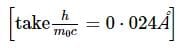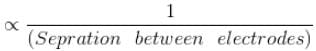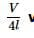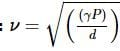NEET  >  AIIMS Physics Mock Test - 10

# AIIMS Physics Mock Test - 10

Test Description

## 60 Questions MCQ Test AIIMS Mock Tests & Previous Year Papers | AIIMS Physics Mock Test - 10

AIIMS Physics Mock Test - 10 for NEET 2022 is part of AIIMS Mock Tests & Previous Year Papers preparation. The AIIMS Physics Mock Test - 10 questions and answers have been prepared according to the NEET exam syllabus.The AIIMS Physics Mock Test - 10 MCQs are made for NEET 2022 Exam. Find important definitions, questions, notes, meanings, examples, exercises, MCQs and online tests for AIIMS Physics Mock Test - 10 below.
Solutions of AIIMS Physics Mock Test - 10 questions in English are available as part of our AIIMS Mock Tests & Previous Year Papers for NEET & AIIMS Physics Mock Test - 10 solutions in Hindi for AIIMS Mock Tests & Previous Year Papers course. Download more important topics, notes, lectures and mock test series for NEET Exam by signing up for free. Attempt AIIMS Physics Mock Test - 10 | 60 questions in 60 minutes | Mock test for NEET preparation | Free important questions MCQ to study AIIMS Mock Tests & Previous Year Papers for NEET Exam | Download free PDF with solutions
 1 Crore+ students have signed up on EduRev. Have you?
AIIMS Physics Mock Test - 10 - Question 1

### A transformer connected to 220 volt line shows an output of 2 A at 11000 volt. The efficiency is 100%. The current drawn from the line is

AIIMS Physics Mock Test - 10 - Question 2

### When wavelength of incident photon is decreased upon

AIIMS Physics Mock Test - 10 - Question 3

### Three resistances each of 4 Ω are connected in the form of an equilateral triangle. The effective resistance between two corners is

AIIMS Physics Mock Test - 10 - Question 4

The weight of a man in a lift moving upward is 608N while the weight of the same man in the lift moving downwards with the same acceleration is 368N. His normal weight in newtons is

AIIMS Physics Mock Test - 10 - Question 5

Monochromatic X - rays of wavelength 0 . 124 Å undergo compton scattering through an angle 60 º from a carbon block. The wavelength of the scatterted X - rays in Å , isAIIMS Physics Mock Test - 10 - Question 6

The energy required to charge a parallel plate condenser of plate separation d and plate area of cross-section A such that the uniform electric field between the plates is E, is

Detailed Solution for AIIMS Physics Mock Test - 10 - Question 6

Energy required = 1 2 CV2 = 1 2 ε0 E2Ad

AIIMS Physics Mock Test - 10 - Question 7

The work functions for sodium and copper are 2 eV and 4 eV. Which of them is suitable for a photocell with 4000Å light

AIIMS Physics Mock Test - 10 - Question 8

When ultraviolet rays are incident on a metal plate, then the photoelectric effect does not occurs. It occurs by the incidence of

AIIMS Physics Mock Test - 10 - Question 9

Consider the following statements regarding the properties of X-rays and electrons :(I) Both ionise gases (II) Both are deflected by electric and magnetic fields (III) Both cause fluorescence in some substances (IV) Both affect the photographic plate. Which of the above statements are correct ?

AIIMS Physics Mock Test - 10 - Question 10

The ratio of secondary to the primary turns in a transformer is 3 : 2. If the power output be P, then the input power, neglecting all losses, must be equal to

AIIMS Physics Mock Test - 10 - Question 11

Two coils are placed close to each other. The mutual inductance of the pair of coils depends upon

AIIMS Physics Mock Test - 10 - Question 12

In an oscillating LC circuit, the maximum charge on the capacitor is Q. The charge on the capacitor, when the energy is stored equally between the electric and magnetic field is

AIIMS Physics Mock Test - 10 - Question 13

Which scientist experimentally proved the existance of electromagnetic waves?

AIIMS Physics Mock Test - 10 - Question 14

The switch in the circuit to the right is initially open. It is closed at time t = 0. Which of the following statements is false?

AIIMS Physics Mock Test - 10 - Question 15

If the distance between the plates of a parallel plate condenser is halved and the dielectric is doubled, then its capacity increases by

AIIMS Physics Mock Test - 10 - Question 16

The region between two concentric spheres of radii R1 and R2 includes a volume charge density given by ρ = a r where a is a constant and r is the distance from the centre. There is a charge Q located at the centre. The value of a for which the region R 1 ≤ r ≤ R 2 has a constant electric field intensity is

AIIMS Physics Mock Test - 10 - Question 17

A long spring is stretched by 2 cm, its potential energy is U. If the spring is stretched by 10 cm, the potential energy stored in it will be

AIIMS Physics Mock Test - 10 - Question 18

A body of mass 2 kg is projected vertically upwards with a velocity of 2 m sec⁻1. The K.E. of the body just before striking the ground is

AIIMS Physics Mock Test - 10 - Question 19

A black body, at a temprature of 227ºC, radiates heat at a rate of 20 cal m⁻2s⁻1. When its temperature is raised to 727ºthe heat radiated by it in cal m⁻2s⁻1 will be closest to

AIIMS Physics Mock Test - 10 - Question 20

The resistance of two bulbs of the same voltage are in the ratio 1:2. On connecting them in parallel the power consumption will be in the ratio

AIIMS Physics Mock Test - 10 - Question 21

The magnetic lines of force inside a bar magnet

AIIMS Physics Mock Test - 10 - Question 22

Isogonic lines are those for which

AIIMS Physics Mock Test - 10 - Question 23

A wire can be broken by applying a load of 200 N.The force required to break another wire of the same length and same material, but double in diameter, is

AIIMS Physics Mock Test - 10 - Question 24

In the above question, the impulse acting on the object is

AIIMS Physics Mock Test - 10 - Question 25

Cobalt = 60 has half life of 5.3 years. The time taken for 7/8 of the original sample to disintegrate will be-

AIIMS Physics Mock Test - 10 - Question 26

A simple pendulum of length 'l' has a maximum angular displacement (θ). The energy of a bob of mass m at the end of the displacement is

AIIMS Physics Mock Test - 10 - Question 27

In the following question, a Statement of Assertion (A) is given followed by a corresponding Reason (R) just below it. Read the Statements carefully and mark the correct answer-
Assertion(A): Thomson's method of determining e m of positive rays, a parabola is obtained on the screen.
Reason(R): Electric field e and magnetic field B are kept parallel to each other.

AIIMS Physics Mock Test - 10 - Question 28

In the following question, a Statement of Assertion (A) is given followed by a corresponding Reason (R) just below it. Read the Statements carefully and mark the correct answer-
Assertion(A): Pressure of a gas in a discharge tube for the production of cathode rays is of the order of 10-1mm of mercury.
Reason(R): At very low pressure, the number of atoms will be too small.

AIIMS Physics Mock Test - 10 - Question 29

In the following question, a Statement of Assertion (A) is given followed by a corresponding Reason (R) just below it. Read the Statements carefully and mark the correct answer-
Assertion(A): The centre of mass of an extended object is that point on the object where the resultant of all the external forces acting on the body acts.
Reason(R) : An object under the effect of several external forces moves as if it were a particle placed at its centre of mass and all forces transferred to the point.

AIIMS Physics Mock Test - 10 - Question 30

In the following question, a Statement of Assertion (A) is given followed by a corresponding Reason (R) just below it. Read the Statements carefully and mark the correct answer-
Assertion(A): The slope of work-time graph gives instantaneous power.
Reason(R): Instantaneous power is dot product of force and displacement.

AIIMS Physics Mock Test - 10 - Question 31

In the following question, a Statement of Assertion (A) is given followed by a corresponding Reason (R) just below it. Read the Statements carefully and mark the correct answer-
Assertion(A): A system cannot have mutual inductance without having self inductances.
Reason(R): If mutual inductance of a system is zero, its self inductances must be zero.

AIIMS Physics Mock Test - 10 - Question 32

In the following question, a Statement of Assertion (A) is given followed by a corresponding Reason (R) just below it. Read the Statements carefully and mark the correct answer-
Assertion(A):A soda water bottle is falling freely the bubbles of the gas will not rise in water.
Reason(R):Pressure in the water does not increase with depth.

AIIMS Physics Mock Test - 10 - Question 33

In the following question, a Statement of Assertion (A) is given followed by a corresponding Reason (R) just below it. Read the Statements carefully and mark the correct answer-
Assertion(A):Penetrating power of β-particles is more compared to α-particles.
Reason(R):Ionising power of β-particles is more compared to α-particles.

AIIMS Physics Mock Test - 10 - Question 34

In the following question, a Statement of Assertion (A) is given followed by a corresponding Reason (R) just below it. Read the Statements carefully and mark the correct answer-
Assertion(A): When wavelength of light is increased, the refractive index of the medium increases.
Reason (R): Refractive index ∝ wavelength of light.

AIIMS Physics Mock Test - 10 - Question 35

In the following question, a Statement of Assertion (A) is given followed by a corresponding Reason (R) just below it. Read the Statements carefully and mark the correct answer-
Assertion(A): The more the sparking potential , the less the separation between the electrodes of a discharge tube.
Reason(R): Sparking potentialAIIMS Physics Mock Test - 10 - Question 36

In the following question, a Statement of Assertion (A) is given followed by a corresponding Reason (R) just below it. Read the Statements carefully and mark the correct answer-
Assertion(A): The potential of a charged conductor throughout its volume is same.
Reason(R): The line integral of electric field is zero along any path.

AIIMS Physics Mock Test - 10 - Question 37

In the following question, a Statement of Assertion (A) is given followed by a corresponding Reason (R) just below it. Read the Statements carefully and mark the correct answer-
Assertion(A): The moment of inertia of a given body about an axis through its centre of mass is smaller than the moment of inertia of the body about any other parallel axis.
Reason(R) : All the force acting on the body can be assumed to act at its centre of mass for its translatory motion.

AIIMS Physics Mock Test - 10 - Question 38

In the following question, a Statement of Assertion (A) is given followed by a corresponding Reason (R) just below it. Read the Statements carefully and mark the correct answer-
Assertion(A): Torque is an axial vector and directed along the axis of rotation.
Reason(R): Torque is equal to vector product of force to the position vector.

AIIMS Physics Mock Test - 10 - Question 39

In the following question, a Statement of Assertion (A) is given followed by a corresponding Reason (R) just below it. Read the Statements carefully and mark the correct answer-
Assertion(A): A planet move faster, when it is closer to the sun in its orbit and vice-versa.
Reason(R): Orbital velocity in an orbit of planet is constant.

AIIMS Physics Mock Test - 10 - Question 40

In the following question, a Statement of Assertion (A) is given followed by a corresponding Reason (R) just below it. Read the Statements carefully and mark the correct answer-
Assertion(A): Steel ball bearings are used in ceiling fans in regulating the speed of rotation of the fan.
Reason(R) : Frictional torques act as a dissipative sources for loss of power in rotation

AIIMS Physics Mock Test - 10 - Question 41

In the following question, a Statement of Assertion (A) is given followed by a corresponding Reason (R) just below it. Read the Statements carefully and mark the correct answer-
Assertion(A): If one of the prongs of a fork is broken, there will be only a forced vibration.
Reason(R): On breaking of a prong, it will only be a rod and so a particular frequency of vibration will not be available unless an external agency continues to vibrate.

AIIMS Physics Mock Test - 10 - Question 42

In the following question, a Statement of Assertion (A) is given followed by a corresponding Reason (R) just below it. Read the Statements carefully and mark the correct answer-
Assertion(A): Diatonic scales have eight notes covering double octave.
Reason(R): Mach number is used to express the speed of objects in relation to the speed of sound.

AIIMS Physics Mock Test - 10 - Question 43

In the following question, a Statement of Assertion (A) is given followed by a corresponding Reason (R) just below it. Read the Statements carefully and mark the correct answer-
Assertion(A): Intensity of sound wave changes when the listener moves towards or away from the stationary source.
Reason(R) : The motion of listener causes the apparent change in wavelength.

AIIMS Physics Mock Test - 10 - Question 44

In the following question, a Statement of Assertion (A) is given followed by a corresponding Reason (R) just below it. Read the Statements carefully and mark the correct answer-
Assertion(A): The ratio of the first three harmonics in a closed pipe bear a ratio 1:3:5.
Reason(R): Frequency is an odd multiple ofwhere l is the length of the pipe.

AIIMS Physics Mock Test - 10 - Question 45

In the following question, a Statement of Assertion (A) is given followed by a corresponding Reason (R) just below it. Read the Statements carefully and mark the correct answer-
Assertion(A): In an elastic collision of two billiard balls, the total kinetic energy is conserved during the short time of oscillation of the balls, when they are in contact.
Reason(R): Energy spent against friction does not follow the law of conservation of energy.

AIIMS Physics Mock Test - 10 - Question 46

In the following question, a Statement of Assertion (A) is given followed by a corresponding Reason (R) just below it. Read the Statements carefully and mark the correct answer-
Assertion(A): A graph between the absolute temperature (T) of a gas and the square of speed of sound (v2) will bea parabola.
Reason(R)AIIMS Physics Mock Test - 10 - Question 47

An electric motor exerts a force of 40 N on a cable and pulls it by a distance of 30 m in one minute. The power supplied by the motor is

AIIMS Physics Mock Test - 10 - Question 48

A bomber plane is moving horizontally with a speed of 500 ms-1 and a bomb released from it, strikes the ground in 10 s. The angle at which it strikes the ground is (g = 10 m/s2)

Detailed Solution for AIIMS Physics Mock Test - 10 - Question 48

Here, νx = 500 m/s; νy = gt = 10 X 10 = 100 m/s
tan θ = νy/ νx = 100/500 = 1/5
or θ = tan−1 1/5

AIIMS Physics Mock Test - 10 - Question 49

The dark lines in the solar spectrum are due to

AIIMS Physics Mock Test - 10 - Question 50

A convex lens of focal length 40 cm is in contact with a concave lens of focal length 25 cm. The power of combination is

AIIMS Physics Mock Test - 10 - Question 51

A symmetric double convex lens is cut in two equal parts by a plane perpendicular to the principal axis. If the power of the original lens is 4D, the power of a cut lens will be

AIIMS Physics Mock Test - 10 - Question 52

In an intrinsic semiconductor the Fermi level is

AIIMS Physics Mock Test - 10 - Question 53

A hole in a p-type semiconductor is

AIIMS Physics Mock Test - 10 - Question 54

The amount of heat produced in a resistor when a current is passed through it can be found using

AIIMS Physics Mock Test - 10 - Question 55

The dimensions of Planck's constant and angular momentum are respectively

AIIMS Physics Mock Test - 10 - Question 56

Identity the pair whose dimensions are equal

AIIMS Physics Mock Test - 10 - Question 57

Who was the first to demonstrate the phenomenon of interference of light?

AIIMS Physics Mock Test - 10 - Question 58

According to wave theory, light is a form of energy which travels through a medium in the form of

AIIMS Physics Mock Test - 10 - Question 59

Both light and sound waves produce diffraction. It is more difficult to observe the diffraction with light waves because

AIIMS Physics Mock Test - 10 - Question 60

A uniform chain of length L and mass M is lying on a smooth table and one third of its length is hanging vertically down over the edge of the table. If g is acceleration due to gravity, the work required to pull the hanging part on to the table is

## AIIMS Mock Tests & Previous Year Papers

3 videos|41 docs|66 tests
 Use Code STAYHOME200 and get INR 200 additional OFF Use Coupon Code
Information about AIIMS Physics Mock Test - 10 Page
In this test you can find the Exam questions for AIIMS Physics Mock Test - 10 solved & explained in the simplest way possible. Besides giving Questions and answers for AIIMS Physics Mock Test - 10, EduRev gives you an ample number of Online tests for practice

## AIIMS Mock Tests & Previous Year Papers

3 videos|41 docs|66 tests

### How to Prepare for NEET

Read our guide to prepare for NEET which is created by Toppers & the best Teachers